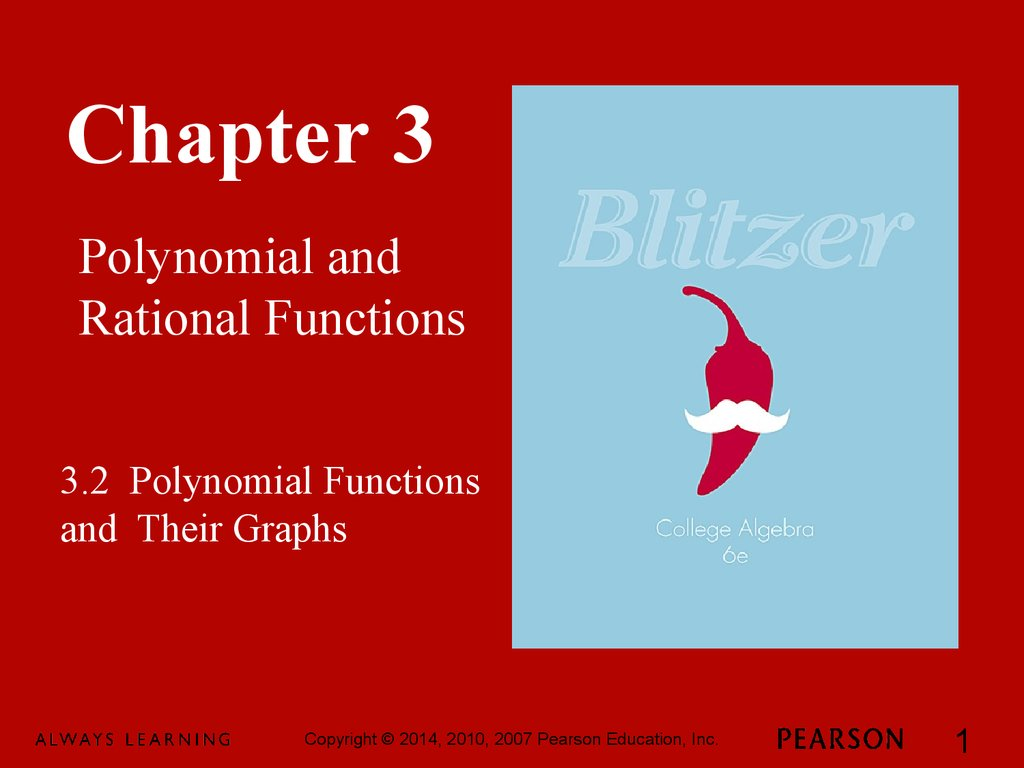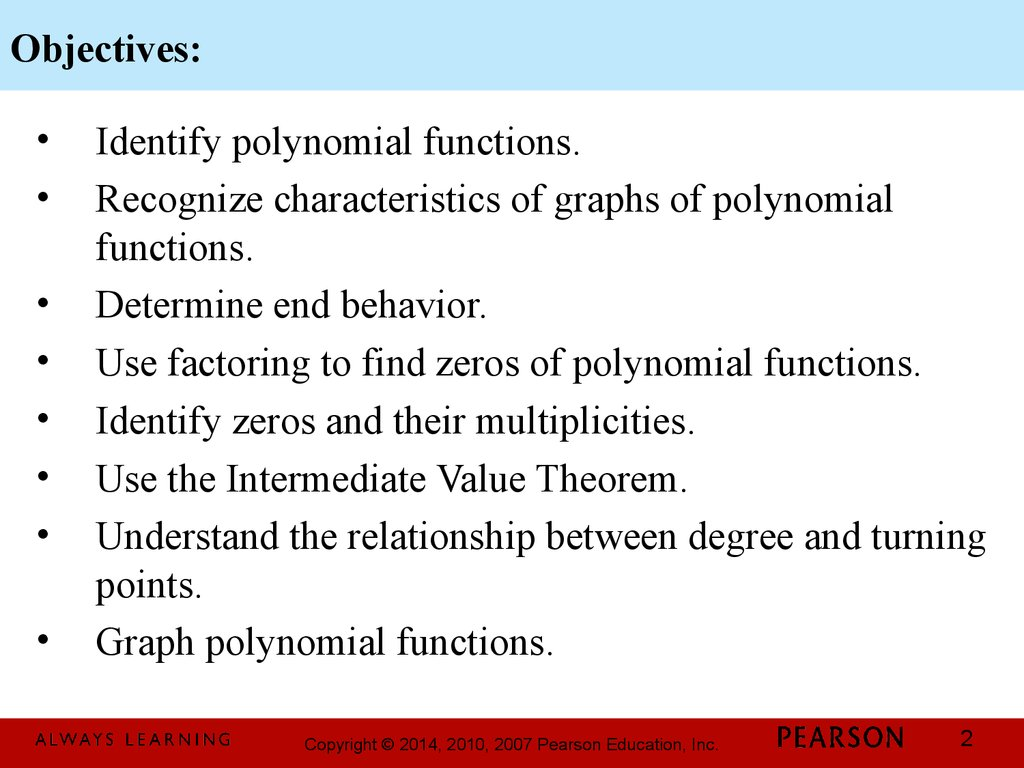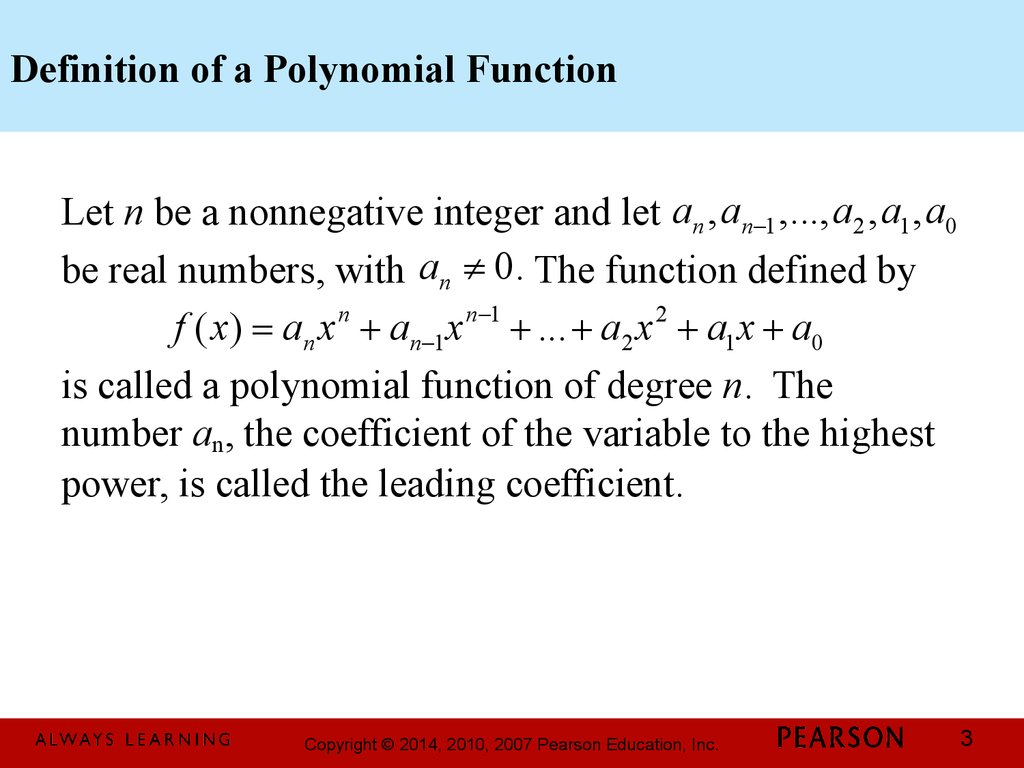# Chapter 3. Polynomial and Rational Functions. 3.2 Polynomial Functions and Their Graphs

## 1.

Chapter 3
Polynomial and
Rational Functions
3.2 Polynomial Functions
and Their Graphs
1

## 2. Objectives:

Identify polynomial functions.
Recognize characteristics of graphs of polynomial
functions.
Determine end behavior.
Use factoring to find zeros of polynomial functions.
Identify zeros and their multiplicities.
Use the Intermediate Value Theorem.
Understand the relationship between degree and turning
points.
Graph polynomial functions.
2

## 3. Definition of a Polynomial Function

Let n be a nonnegative integer and let an , an-1 ,..., a2 , a1 , a0
be real numbers, with an ¹ 0. The function defined by
f ( x) = an x n + an-1 x n-1 + ... + a2 x 2 + a1 x + a0
is called a polynomial function of degree n. The
number an, the coefficient of the variable to the highest
power, is called the leading coefficient.
3

## 4. Graphs of Polynomial Functions – Smooth and Continuous

Polynomial functions of degree 2 or higher have graphs
that are smooth and continuous.
By smooth, we mean that the graphs contain only
rounded curves with no sharp corners.
By continuous, we mean that the graphs have no breaks
and can be drawn without lifting your pencil from the
rectangular coordinate system.
4

## 5. End Behavior of Polynomial Functions

The end behavior of the graph of a function to the far
left or the far right is called its end behavior.
Although the graph of a polynomial function may
have intervals where it increases or decreases, the graph
will eventually rise or fall without bound as it moves far
to the left or far to the right.
The sign of the leading coefficient, an, and the
degree, n, of the polynomial function reveal its end
behavior.
5

## 6. The Leading Coefficient Test

As x increases or decreases without bound, the graph of
the polynomial function
f ( x) = an x n + an-1 x n-1 + ... + a2 x 2 + a1x + a0
eventually rises or falls. In particular, the sign of the
leading coefficient, an, and the degree, n, of the
polynomial function reveal its end behavior.
6

## 7. The Leading Coefficient Test for (continued)

f ( x) = an x n + an-1 x n-1 + ... + a2 x 2 + a1x + a0 (continued)
7

## 8. Example: Using the Leading Coefficient Test

Use the Leading Coefficient Test to determine the end
4
2
behavior of the graph of f ( x) = x - 4 x
The degree of the function is 4,
which is even. Even-degree
functions have graphs with the
same behavior at each end.
positive. The graph rises to
the left and to the right.
8

## 9. Zeros of Polynomial Functions

If f is a polynomial function, then the values of x for
which f(x) is equal to 0 are called the zeros of f.
These values of x are the roots, or solutions, of the
polynomial equation f(x) = 0.
Each real root of the polynomial equation appears as an
x-intercept of the graph of the polynomial function.
9

## 10. Example: Finding Zeros of a Polynomial Function

3
2
f
(
x
)
=
x
+
2
x
- 4x - 8
Find all zeros of
We find the zeros of f by setting f(x) equal to 0 and
solving the resulting equation.
f ( x) = x3 + 2 x 2 - 4 x - 8
0 = x3 + 2 x 2 - 4 x - 8
0 = x 2 ( x + 2) - 4( x + 2)
0 = ( x + 2)( x 2 - 4)
( x + 2) = 0 or ( x 2 - 4) = 0
x+2=0
x = -2
x2 - 4 = 0
x2 = 4
x = ± 4 = ±2
10

## 11. Example: Finding Zeros of a Polynomial Function (continued)

Find all zeros of f ( x) = x + 2 x - 4 x - 8
3
The zeros of f are
–2 and 2.
The graph of f shows that
each zero is an x-intercept.
The graph passes through
(0, –2)
and (0, 2).
2
(0, –2)
(0, 2)
11

## 12. Multiplicity and x-Intercepts

If r is a zero of even multiplicity, then the graph
touches the x-axis and turns around at r. If r is a zero of
odd multiplicity, then the graph crosses the x-axis at r.
Regardless of whether the multiplicity of a zero is even
or odd, graphs tend to flatten out near zeros with
multiplicity greater than one.
12

## 13. Example: Finding Zeros and Their Multiplicities

2

æ
3
Find the zeros of f ( x) = -4 ç x + ÷ ( x - 5)

è
and give the multiplicities of each zero. State whether
the graph crosses the x-axis or touches the x-axis and
turns around at each zero.
13

## 14. Example: Finding Zeros and Their Multiplicities (continued)

We find the zeros of f by setting f(x) equal to 0:
2
2

æ
æ
3
f ( x) = -4 ç x + ÷ ( x - 5) ® -4 ç x + ÷ ( x - 5)3 = 0

è
è
2
æ x + 1ö = 0 ® x = -1
3
( x - 5) = 0 ® x = 5
ç
÷

2
è
1
x=2
is a zero of
multiplicity 2.
x = 5 is a zero of
multiplicity 3.
14

## 15. Example: Finding Zeros and Their Multiplicities (continued)

2

æ
3
For the function f ( x) = -4 ç x + ÷ ( x - 5)

è
1 is a zero of
x=x = 5 is a zero of
2 multiplicity 2.
multiplicity 3.
The graph will
touch the
1
x-axis at x = 2
The graph will
cross the
x-axis at x = 5
15

## 16. The Intermediate Value Theorem

Let f be a polynomial function with real coefficients. If
f(a) and f(b) have opposite signs, then there is at least
one value of c between a and b for which f(c) = 0.
Equivalently, the equation f(x) = 0 has at least one real
root between a and b.
16

## 17. Example: Using the Intermediate Value Theorem

Show that the polynomial function f ( x) = 3 x - 10 x + 9
has a real zero between –3 and –2.
We evaluate f at –3 and –2. If f(–3) and f(–2) have
opposite signs, then there is at least one real zero
between –3 and –2.
3
f ( x) = 3 x 3 - 10 x + 9
f (-3) = 3(-3)3 - 10(-3) + 9 = -81 + 30 + 9 = -42
f (-2) = 3(-2)3 - 10(-2) + 9 = -24 + 20 + 9 = 5
17

## 18. Example: Using the Intermediate Value Theorem (continued)

For f ( x) = 3x 3 - 10 x + 9,
f(–3) = –42
(–2, 5)
and f(–2) = 5.
The sign change shows
that the polynomial
function has a real zero
between –3 and –2.
(–3, –42)
18

## 19. Turning Points of Polynomial Functions

In general, if f is a polynomial function of degree n,
then the graph of f has at most n – 1 turning points.
19

20

## 21. Example: Graphing a Polynomial Function

Use the five-step strategy to graph f ( x) = 2( x + 2) ( x - 3)
Step 1 Determine end behavior
Identify the sign of an, the leading coefficient, and the
degree, n, of the polynomial function.
an = 2 and n = 3
2
The degree, 3, is odd. The leading
coefficient, 2, is a positive number.
The graph will rise on the right and
fall on the left.
21

## 22. Example: Graphing a Polynomial Function (continued)

Use the five-step strategy to graph f ( x) = 2( x + 2) ( x - 3)
Step 2 Find x-intercepts (zeros of the function) by
setting f(x) = 0.
2
f ( x) = 2( x + 2) 2 ( x - 3)
x + 2 = 0 ® x = -2
2( x + 2) 2 ( x - 3) = 0
x-3= 0 ® x = 3
x = –2 is a zero of multiplicity 2.
x = 3 is a zero of multiplicity 1.
22

## 23. Example: Graphing a Polynomial Function (continued)

Use the five-step strategy to graph f ( x) = 2( x + 2) ( x - 3)
Step 2 (continued) Find x-intercepts (zeros of the
function) by setting f(x) = 0.
x = –2 is a zero of multiplicity 2.
x=3
x = –2
The graph touches the x-axis
at x = –2, flattens and turns around.
x = 3 is a zero of multiplicity 1.
The graph crosses the x-axis
at x = 3.
2
23

## 24. Example: Graphing a Polynomial Function (continued)

Use the five-step strategy to graph f ( x) = 2( x + 2) ( x - 3)
Step 3 Find the y-intercept by computing f(0).
f ( x) = 2( x + 2) 2 ( x - 3)
2
f (0) = 2(0 + 2) (0 - 3) = 2(4)( -3) = -24
The y-intercept is –24.
The graph passes through the
y-axis at (0, –24).
To help us determine how to scale
the graph, we will evaluate f(x) at x = 1 and x = 2.
2
24

## 25. Example: Graphing a Polynomial Function (continued)

Use the five-step strategy to graph f ( x) = 2( x + 2) ( x - 3)
Step 3 (continued) Find the y-intercept by
computing f(0).
The y-intercept is –24. The graph passes through
the y-axis at (0, –24). To help us determine how to scale
the graph, we will evaluate f(x) at x = 1 and x = 2.
f ( x) = 2( x + 2) 2 ( x - 3)
2
f (1) = 2(1 + 2) 2 (1 - 3) = 2(9)(-2) = -36
f (2) = 2(2 + 2) 2 (2 - 3) = 2(16)(-1) = -32
25

## 26. Example: Graphing a Polynomial Function (continued)

Use the five-step strategy to graph f ( x) = 2( x + 2) ( x - 3)
Step 4 Use possible symmetry to help draw the
graph.
Our partial graph illustrates
that we have neither y-axis
symmetry nor origin symmetry.
2
26

## 27. Example: Graphing a Polynomial Function (continued)

Use the five-step strategy to graph f ( x) = 2( x + 2) ( x - 3)
Step 4 (continued) Use possible symmetry to help
draw the graph.
Our partial graph illustrated
that we have neither y-axis
symmetry nor origin symmetry.
Using end behavior, intercepts,
graph the function.
2
27

## 28. Example: Graphing a Polynomial Function (continued)

Use the five-step strategy to graph f ( x) = 2( x + 2) ( x - 3)
Step 5 Use the fact that the maximum number of
turning points of the graph is n-1 to check whether it
is drawn correctly.
The degree is 3. The maximum
number of turning points will
be 3 – 1 or 2. Because the graph
has two turning points, we have not
violated the maximum number possible.
2